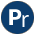#occurs.pl -- Finding and counting sub-terms

This is a SWI-Prolog implementation of the corresponding Quintus library, based on the generalised arg/3 predicate of SWI-Prolog.

- library(terms) provides similar predicates and is probably more wide-spread than this library.
contains_term(+Sub, +Term) is semidet
Succeeds if Sub is contained in Term (=, deterministically)
contains_var(+Sub, +Term) is det
Succeeds if Sub is contained in Term (==, deterministically)
free_of_term(+Sub, +Term)
Succeeds of Sub does not unify to any subterm of Term
free_of_var(+Sub, +Term)
Succeeds of Sub is not equal (==) to any subterm of Term
occurrences_of_term(+SubTerm, +Term, ?Count)
Count the number of SubTerms in Term
occurrences_of_var(+SubTerm, +Term, ?Count)
Count the number of SubTerms in Term
sub_term(-Sub, +Term)
Generates (on backtracking) all subterms of Term.
sub_var(-Sub, +Term)
Generates (on backtracking) all subterms (==) of Term.
sub_term_shared_variables(+Sub, +Term, -Vars) is det
If Sub is a sub term of Term, Vars is bound to the list of variables in Sub that also appear outside Sub in Term. Note that if Sub appears twice in Term, its variables are all considered shared.

An example use-case is refactoring a large clause body by introducing intermediate predicates. This predicate can be used to find the arguments that must be passed to the new predicate.

term_replace_(+From, +To, +TermIn, -TermOut, +Done)[private]
Replace instances (==/2) of From inside TermIn by To.
intersection_eq(+Small, +Big, -Shared) is det[private]
Shared are the variables in Small that also appear in Big. The variables in Shared are in the same order as Small.
count(:Goal, -Count)[private]
Count number of times Goal succeeds.